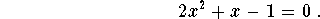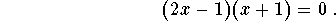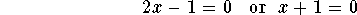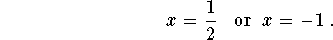#Quadratic Equations: Solutions by FactoringSometimes it is easier to find solutions or roots of a quadratic equation by factoring. Indeed, the basic principle to be used is:
if a and b are real or complex numbers such that ab=0, then a=0 or b=0 .

Example : Solve the equationSolution. Factoring yields the equation:Hence we havewhich yield[Algebra] [Complex Variables]
[Geometry] [Trigonometry ]
[Calculus] [Differential Equations] [Matrix Algebra]S.O.S MATHematics home page

Do you need more help? Please post your question on our S.O.S. Mathematics CyberBoard.Author: Mohamed Amine Khamsi